### 2. METHOD OF SOLUTION

2.1. The equations of statistical equilibrium

In order to calculate the level populations of a given atom or ion, we make two basic assumptions :

1. The rates of processes involving ionization stages other than the atom or ion being considered (such as direct photoionization or recombination, charge exchange reactions, collisional ionization, etc.) are slow compared to bound-bound rates.
2. All transitions considered are optically thin.

To calculate the population of some level i, we must take into account all possible processes that will (de)populate it:(1)

where ni is the volume density of atoms or ions in level i.

Therefore, in steady state regime the sum over all processes that populate level i will be balanced by the sum over all processes that depopulate level i. Assuming that the two conditions listed above are met, this can be written (see, for instance, Rybicki and Lightman ):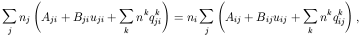(2)

where we have considered all possible bound-bound processes, i.e., spontaneous, radiation-induced and collisionally-induced. The lefthand side of eq. (2) is the sum over all processes that populate level i from the other levels j, whereas the righthand side is the sum over all processes that depopulate level i to levels j.

Aij is the transition probability of spontaneous decay from level i to level j. For ij, Aij = 0.

Bij are Einstein coefficients, related to the transition probabilities by: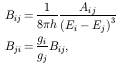(3)

for i > j, and Bii = 0; h is Plank's constant, Ei is the energy of level i (expressed in cm-1) and gi is the statistical weight of level i.

uij is the spectral energy density of the radiation field integrated along the line profile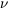of the transition from level i to level j: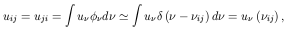(4)

with uii = 0;ij is the frequency of the transition and we have assumed that the radiation field does not vary significantly along the line profile.

In eq. (2) we have also considered the effect of collisions; nk is the volume density of the particle inducing the transition, the main collision partners usually being k = e-, p+, H0, He0, H2,... , depending whether the medium is primarily ionized or neutral.

qkij is the collision rate for the transition from level i to level j induced by the collision partner k . These coefficients are the cross-sections for the related processij convolved with a Maxwellian distribution of velocities f(v), making these quantities suitable for astrophysical applications (see, for instance, Osterbrock ):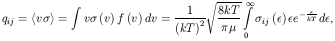(5)

for the deexcitation rates (i > j); k is Boltzmann's constant, T is the kinetic temperature, µ is the reduced mass of the system andis the collision partner's kinetic energy.

Excitation and deexcitation rates are related by the principle of detailed balance: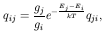(6)

with qii = 0. When the interaction is coulombian, as in collisions with electrons, it is convenient to express the cross-section in terms of the collision stregthij, defined by: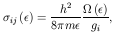(7)

where m is the electron's mass. Substituting this in eq. (5) yields: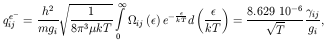(8)

with T expressed in K andij is defined by the integral in eq. (8) and is called Maxwellian-averaged collision stregth. Typicallyij is a slowly varying function of T, of order unity. However, for neutral atoms it may vary for several orders of magnitude.

These are the basic parameters needed to solve eq. (2). If we consider our model ion to be composed of n levels, then we must solve a linear system of n - 1 equations in order to calculate the relative population ratios.